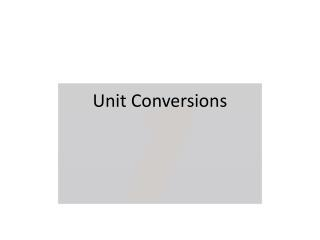# Unit Conversions - PowerPoint PPT PresentationDownload PresentationUnit Conversions

Unit ConversionsDownload Presentation## Unit Conversions

- - - - - - - - - - - - - - - - - - - - - - - - - - - E N D - - - - - - - - - - - - - - - - - - - - - - - - - - -
##### Presentation Transcript

1. Unit Conversions

2. Objective • Convert between different SI units • Convert English units to SI units

3. Real World Example • Mars climate orbiter in 1999 • Engineers at Lockheed Martin using US system for units • Engineers at NASA using SI system for units  Led to explosion of the Mars orbiter and loss of \$125 million

4. Agenda • Do Now • Heart of the Lesson #1 – Dimensional Analysis • Summary • HW: 1.11 HW Dimensional Analysis + review questions Get gradesheet signed Quiz tomorrow

5. Conversion factors…in our lives

6. Conversion Factor: • Factor equal to 1 • Use it to convert from one unit to another

7. Dimensional Analysis: • Problem-solving method that uses conversion factors to convert between units • Not changing the value of the measurement, only the units

8. BIG IDEA #1 for Dimensional Analysis • 4/4 = 1 • 10/10 = 1 • x/x = 1 • BIG IDEA #1: any value divided by itself = 1

9. Example 24 hours = 1 day = = 1 The conversion factor is equal to 1 (any value divided by itself = 1)

10. BIG IDEA #2 for Dimensional Analysis • 4 x1 = 4 • 10 x 1 = 10 • y x 1 = y • BIG IDEA #2: any value multiplied by 1 has the same value

11. Example #1 How many centimeters are in 1 meter? 100 Definition of a centimeter: 1 cm = 10-2 m Conversion factor: 102 cm = 1 m 100 cm = 1 meter

12. How many centimeters are in 3 meters? • Are centimeters bigger or smaller than meters? Smaller • For any number of meters, I will have more centimeters • So in 3 meters, will I have more or less than 3 centimeters? More

13. For any number of meters, I will have more centimeters • Since I want the final number to bebigger, I have to multiply the original measurement by the conversion factor

14. Conversion factor: Multiply orig. measurement by the conversion factor: 3 m x

15. 3 m x 3 m x 3 x 100 cm = 300 cm There are 300 cm in 3 meters

16. Example #2: How many hours are equivalent to 250 seconds? Conversion factors: 60 min = 1 hr 60 sec = 1 min • Are hours bigger or smaller than seconds? Bigger  For any number of seconds, I will have fewer hours • Since I want the final number to be smaller, I have to divide the original measurement by the conversion factor

17. Divide by the conversion factors: and 250 s x x

18. 250 s x x 250 s x x hr = .0694 hr There are .0694 hours in 250 seconds

19. Summary • If you want the final answer to be bigger, you multiply by the conversion factor • If you want the final answer to be smaller, you divide by the conversion factor • The value is the same, but the units change • Conversion factor is what you use to convert from one unit to another • Dimensional analysis is a way to convert btwn 2 diff units

20. Gradesheets • Grading Policy • Quizzes – 25% • Interim Assessments – 20% • Tests – 15% • Homework – 15% • Classwork – 10% • Labs & Projects – 10% • Participation – 5%